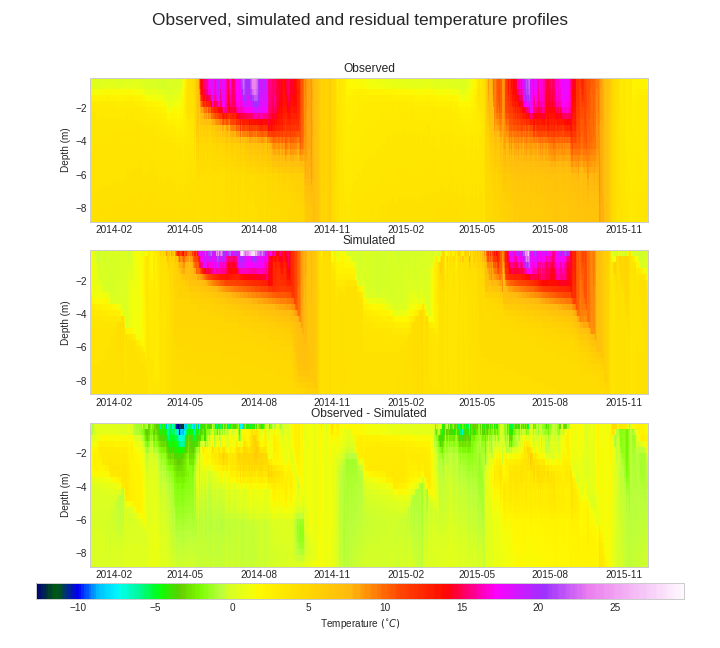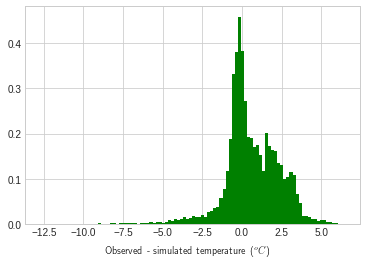In :
%matplotlib inline
#import pygments
#from pygments import highlight
#from pygments.lexers import MakefileLexer, CppLexer
#from pygments.formatters import HtmlFormatter
from IPython.core.display import display,HTML

import sys
print(sys.version, sys.path)

import sqlite3
import xml.etree.ElementTree as ET
import ruamel.yaml as yaml
import numpy as np
import matplotlib
import matplotlib.pyplot as plt
from IPython.display import Image
from matplotlib.ticker import FormatStrFormatter
import os
import fileinput
import re
import subprocess
from displayNC import displayVariables,temperatureDisplay, getSingleArray
from netCDF4 import Dataset
from scipy import stats
import datetime
from plotly import __version__
import plotly.graph_objs as go
import itertools
import pandas as pd

init_notebook_mode(connected=True)

plt.style.use('seaborn-whitegrid')

def plot_dotty(param,perf,names,figname) :
num_rows = len(names)
fig, axes = plt.subplots(nrows = num_rows, ncols = 1, figsize = (10, num_rows * 2.5))
cnt = 0
formatter = matplotlib.ticker.ScalarFormatter(useOffset=False)
for name,aH in zip(names, axes) :
plt.sca(aH)
lH, = plt.plot(param[:,cnt],perf,'b.',markersize = 1)
aH.set_xlabel(name)
#print name
#plt.xlim = (-100000,-70000)  #This is hardcoded and is horrible. Will eventually fail
aH.yaxis.set_major_formatter(formatter)
aH.xaxis.set_major_formatter(formatter)
aH.locator_params(axis='y',tight=True,nbins=6)
cnt = cnt + 1
fig.tight_layout()
#plt.savefig(figname)
plt.close()

def setInYaml(cur, list, value):
if len(list) == 1:
cur[list] = value
return
if not list in cur:
cur[list] = {}
setInYaml(cur[list], list[1:], value)

3.5.2 (default, Nov 23 2017, 16:37:01)
[GCC 5.4.0 20160609] ['', '/usr/lib/python35.zip', '/usr/lib/python3.5', '/usr/lib/python3.5/plat-x86_64-linux-gnu', '/usr/lib/python3.5/lib-dynload', '/usr/local/lib/python3.5/dist-packages', '/usr/local/lib/python3.5/dist-packages/onedrive_d-1.1.0.dev0-py3.5.egg', '/usr/local/lib/python3.5/dist-packages/daemonocle-1.0.1-py3.5.egg', '/usr/local/lib/python3.5/dist-packages/click-6.6-py3.5.egg', '/usr/lib/python3/dist-packages', '/usr/local/lib/python3.5/dist-packages/IPython/extensions', '/home/jose-luis/.ipython']


# Setup of the GOTM/FABM model for Langtjern¶

## Compiling GOTM and FABM¶

Please follow the instructions at https://github.com/gotm-model/code. Please note that the provided instructions are only available for Linux systems at present.

For Windows, a combination of Visual Studio, CMake and the Intel Fortran compiler should work with minimal modifications when CMake is setup to generate Visual Studio project files.

For the rest of this document, it is assumed that Linux is used in combination with CMake, using GCC-5.4.

### Getting the source code¶

Credentials might be required for repositories. The text highlighted in a box should be run in a shell.

#### GOTM¶

Clone the source code to a directory of your choice.

git clone https://github.com/gotm-model/code.git

Switch to lake branch.

git checkout lake

#### FABM¶

Clone the source code to a directory of your choice.

git clone [email protected]:anbios/fabm-prognos.git

#### Langtjern setup¶

Clone the source code to a directory of your choice.

git clone [email protected]:anbios/PROGNOS.git

### Compiling¶

#### FABM¶

From within FABM source directory.

Out-of-source builds should be preferred. Neater. Create a ./build directory in the root folder for FABM (or wherever you like but point to the right /src folder in the cmake command below:

mkdir build
cd build
cmake ../src -DFABM_HOST=gotm
make
make install

Note that make will create libfabm.a in the .build directory. make install will place the library and some other include files in the ~/local directory. The later is convenient to have all the builds for both FABM and GOTM in a single directory (that can be added to the PATH) thus simplifying linking.

#### GOTM¶

From within GOTM source directory.

Repeating the comments for the FABM compilation. Create a ./build directory in the root folder for GOTM. You will need to point cmake to FABM root dir. Modify the cmake command below to point the the right locations if necessary:

mkdir build
cd build
cmake ../src -DFABM_BASE=../../fabm-gotm
make
make install

### Running GOTM from the "PROGNOS/Langtjern" folder.¶

GOTM and FABM can take a variety of inputs, depending on data availability. The format required is described in the FABM repository. In short, model setup relies on three different types of files:

1. .xml General setup file. The different settings in .nml files (see next item) will be read from the langtjern.xml file inside the "Langtjern" folder.
2. .nml (nameless) files, to regulate the interaction between FABM and GOTM. And also to specify processes to be used within GOTM.
3. .yaml to specify which biogeochemical models will be used and their parameterizations.
4. .dat data files used to force and evaluate the model. They are in the format specified in the above link. Their are two basic flavors of data files: depth-dependent or independent.

In order to start running simulations with GOTM and FABM, some basic setup needs to be peformed first.

From the "Langtjern" folder in the PROGNOS repository, editscenario.sh —a wrapper to a python script— needs to be run. This basically creates the gotm_fabm.nml and other nameless files in the "Langtjern" folder, filling with options read from the Langtjern.xml file.

Important note: The default option is NOT to use FABM when running GOTM. In order to change this fabm_calc needs to be set to .true. in gotm_fabm.nml.

If you want to modify any setting there are two options:

1. Modify the Langtjern.xml file and run editscenario.sh.
2. Modify the nameless files.

#### Setting up the meteo data¶

In order to run GOTM some basic meteo data are required. In this case, the file is called meteo_file.dat and should be located inside the Langtjern folder. When instantiated through editscenario.sh this file while be a copy of the file specified in langtjern.xml. The file telling GOTM where the meteo data are located is airsea.nml.

In our particular setup, the meteo file was generated by running a Python jupyter notebook that loads data from AquaMonitor and puts in in the format required by GOTM/FABM.

#### Running GOTM¶

The Langtjern.xml file was modified to point to file generated by the Jupyter notebook that processes the AquaMonitor data.

After running "./editscenario.sh" the meteo data should be in place and GOTM should run.

./gotm

### Calibrating GOTM¶

ACPY, a python package developped by B&B should be installed first with the provided wheel file.

Once ACPY is installed and on the path, it should be run from the ACPY folder in the Langtjern folder.

acpy run config_acpy.xml

A temp.obs file containing the meteo data should be present in the ACPY folder. Note that the format is slightly different than the one required by FABM/GOTM.

#### Results¶

All the parameters tested during the calibration are saved in a sqlite database, whose name is specified in config_acpy.xml. Let's visualize the results of the calibration.

In :
basePath  = '/home/jose-luis/Dropbox/NIVA/PROGNOS_Development/PROGNOS/langtjern/acpy_feb01_physical_model/'
configFile = 'config_acpy_fabm.xml'

#Getting name of database from config_acpy.xml
tree = ET.parse(basePath + configFile)
root = tree.getroot()
p = tree.find("transports")
db_element = p.find("transport")
db_element = db_element.attrib
#db_path = basePath + db_element["path"]
db_path = basePath + db_element["path"]

#Getting parameter names from config_acpy.xml
p = tree.find("parameters")
cnt = 0
variable_list = []
for i in p :
cnt += 1
dummy = i.attrib
if 'variable' in dummy :
variable_list.append(dummy["variable"])
else :
variable_list.append("dummy")

print("The variables being calibrated are: ", variable_list)
print(db_path)

The variables being calibrated are:  ['shf_factor', 'swr_factor', 'wind_factor', 'k_min', 'A', 'g1', 'g2', 'instances/dom/parameters/kOM2', 'instances/dom/parameters/kOM1', 'instances/dom/parameters/Kin_O2', 'instances/dom/parameters/Km_NO3', 'instances/dom/parameters/theta', 'instances/dom/parameters/k_floc']
/home/jose-luis/Dropbox/NIVA/PROGNOS_Development/PROGNOS/langtjern/acpy_feb01_physical_model/langtjern_fabm.db

In :
#Open database connection
print(db_path)
conn = sqlite3.connect(db_path)
c = conn.cursor()
#Getting the "best" parameter set according to the log-likelihood
c.execute("SELECT parameters,lnlikelihood FROM results WHERE lnlikelihood NOT NULL AND run=1 ORDER BY lnlikelihood DESC LIMIT 1")
params = c.fetchone()
log_like = float(params)
params = [float (x) for x in params.split(';')]

print("The best performing parameters are: ")
for a,b in zip(variable_list,params) :
print (a, "\t->\t ", b)
print("With log-likelihood -> ", log_like)
conn.close()

/home/jose-luis/Dropbox/NIVA/PROGNOS_Development/PROGNOS/langtjern/acpy_feb01_physical_model/langtjern_fabm.db
The best performing parameters are:
shf_factor 	->	  1.457009280759171
swr_factor 	->	  1.353466062576801
wind_factor 	->	  0.9735023146527344
k_min 	->	  1.27299761177284e-09
A 	->	  0.01588218525227281
g1 	->	  0.4961775545303269
g2 	->	  28.32494371860784
instances/dom/parameters/kOM2 	->	  0.1288991588519032
instances/dom/parameters/kOM1 	->	  0.8993528976004547
instances/dom/parameters/Kin_O2 	->	  10447664.79697201
instances/dom/parameters/Km_NO3 	->	  5587186.39885591
instances/dom/parameters/theta 	->	  1.487088229849371
instances/dom/parameters/k_floc 	->	  8.81973218135659e-14
With log-likelihood ->  -29614.734720056098

In :
#Dotty plots simulation performed during calibration
#Storing the parameters as a numpy array
conn = sqlite3.connect(db_path)
c = conn.cursor()
c.execute("SELECT parameters,lnlikelihood FROM results WHERE lnlikelihood NOT NULL ORDER BY lnlikelihood DESC");
dotty_data = c.fetchall()
param_array = np.zeros((len(dotty_data), len(params)))
perf_array = np.zeros((len(dotty_data)))
cnt = 0
for i in dotty_data :
#print(i)
param_array[cnt][:] = np.fromstring(i,sep=';')
perf_array[cnt] = float(i)
cnt = cnt + 1

num_dots = int(len(perf_array)*0.5)
plot_dotty(param_array[:num_dots,:],perf_array[:num_dots],variable_list,"dotty.jpg")
del param_array, perf_array
conn.close()

Image(filename='dotty.jpg')

Out:#### Running GOTM/FABM with the best performing parameter set¶

Setting parameter values in the .nml and .yaml files. The list of files is read from an acpy configuration file, e.g. config_acpy.xml. The values to be set were previously obtained from the acpy result database, selecting the parameter set with the best lnlikelihood.

In :
#Loading parameter file information from config_acpy.xml
tree = ET.parse(basePath + configFile)
root = tree.getroot()
p = tree.find("parameters")
parentPath = os.path.realpath(os.path.join(basePath,'..'))

#Replacing paramters in file
#This is laughingly inefficient but should do for smallish nml files
print("Setting parameter value in file:")
for child, value in zip(p,params):
db_element = child.attrib
if 'file' in db_element :
_,ext = os.path.splitext(db_element['file'])
filename = parentPath + '/' + db_element['file']
pattern = db_element['variable'] + '\s*=.*$' print(filename) if ext == ".nml" : replacement = db_element['variable'] + ' = ' + str(value) + ',' cnt = 0 f = fileinput.FileInput(filename, inplace=True) for line in f : line = re.sub(pattern,replacement,line.rstrip()) print(line) f.close() if ext == ".yaml" : strMap = db_element["variable"].split('/') with open(filename, 'r') as f: yamlData = yaml.round_trip_load(f) f.close() setInYaml(yamlData,strMap,value) with open(filename, "w") as f: yaml.round_trip_dump(yamlData,f,default_flow_style=False) f.close()  Setting parameter value in file: /home/jose-luis/Dropbox/NIVA/PROGNOS_Development/PROGNOS/langtjern/airsea.nml /home/jose-luis/Dropbox/NIVA/PROGNOS_Development/PROGNOS/langtjern/airsea.nml /home/jose-luis/Dropbox/NIVA/PROGNOS_Development/PROGNOS/langtjern/airsea.nml /home/jose-luis/Dropbox/NIVA/PROGNOS_Development/PROGNOS/langtjern/gotmturb.nml /home/jose-luis/Dropbox/NIVA/PROGNOS_Development/PROGNOS/langtjern/obs.nml /home/jose-luis/Dropbox/NIVA/PROGNOS_Development/PROGNOS/langtjern/obs.nml /home/jose-luis/Dropbox/NIVA/PROGNOS_Development/PROGNOS/langtjern/obs.nml /home/jose-luis/Dropbox/NIVA/PROGNOS_Development/PROGNOS/langtjern/fabm.yaml /home/jose-luis/Dropbox/NIVA/PROGNOS_Development/PROGNOS/langtjern/fabm.yaml /home/jose-luis/Dropbox/NIVA/PROGNOS_Development/PROGNOS/langtjern/fabm.yaml /home/jose-luis/Dropbox/NIVA/PROGNOS_Development/PROGNOS/langtjern/fabm.yaml /home/jose-luis/Dropbox/NIVA/PROGNOS_Development/PROGNOS/langtjern/fabm.yaml /home/jose-luis/Dropbox/NIVA/PROGNOS_Development/PROGNOS/langtjern/fabm.yaml  In : #And now running gotm callStr = parentPath + '/' + 'gotm' print(parentPath) p = subprocess.Popen('./gotm', cwd = parentPath) p.wait()  /home/jose-luis/Dropbox/NIVA/PROGNOS_Development/PROGNOS/langtjern  Out: 0 #### Evaluating results¶ Loading the observed data: In : filename = parentPath + '/output.nc' var_to_show = ['tprof','temp'] fig_name = "langtjern_temperatures.png" temperatureDisplay(filename,fig_name) Image(filename=fig_name)  -12.7467 29.483  Out:And showing some statistics for the residuals: In :  nc = Dataset(filename, mode='r') obs = nc.variables['tprof'][:] obs = np.flipud(np.transpose(np.squeeze(obs))) sim = nc.variables['temp'][:] sim = np.flipud(np.transpose(np.squeeze(sim))) zz = np.flipud(np.array(nc.variables['z'])) tt = nc.variables['time'] startTime = datetime.datetime.strptime(tt.units,'seconds since %Y-%m-%d %H:%M:%S') timestamp = np.squeeze(np.array(nc.variables['time'][:])) timestamp = [(startTime + datetime.timedelta(seconds = x)) for x in timestamp] nc.close() residuals = obs-sim; #fig = plt.plot(residuals.flatten(),'k-', markersize = 1) fig = plt.hist(residuals.flatten(), 100, normed=1,facecolor='green') #plt.show() #plt.tight_layout() plt.xlabel('Observed - simulated temperature ($^{o}C$)',usetex=True) plt.savefig('residuals.png') print(stats.describe(residuals.flatten())) #Various statistics print('MAE',np.mean(np.absolute(residuals.flatten()))) print('NSE', 1 - np.sum((residuals.flatten())**2) / np.sum((obs.flatten()-np.mean(obs.flatten()))**2) ) print('RMSE', np.sqrt(np.mean(residuals.flatten()**2)))  DescribeResult(nobs=17500, minmax=(-12.746735, 6.446125), mean=0.59135014, variance=2.9184358, skewness=-0.598031222820282, kurtosis=3.3445505965118034) MAE 1.31287 NSE 0.782729297876 RMSE 1.80775Also showing modelling results by depth: In : data = [] for a,b,dd in zip(obs,sim,zz) : dummy = dict( visible = False, line=dict(color='blue', width = 1), x = timestamp, y = a, name = 'Observed temperature, depth = ' + ("%4.2f m" % (dd)) ) dummy0 = dict( visible = False, line=dict(color='red', width = 1), x = timestamp, y = b, mode = 'markers', name = 'Simulated temperature, depth = ' + ("%4.2f m" % (dd)) ) data.append([dummy,dummy0]) for i in data[:] : i['visible'] = True flattenList = lambda x : list(itertools.chain(*x)) layout = go.Layout(title='Obs. and Sim. temperature at different depths', yaxis=dict(range=(-2,35)), legend =dict(orientation = "h")) fig = go.Figure(data=flattenList(data),layout=layout) #iplot(fig, filename='dummy.html') steps = [] #getting tempStr is farcically convoluted for i in range(len(data)) : tempStr = data[i]['name'] m = re.search('[0-9.]+\sm$',tempStr)
tempStr = m.group(0)
step = dict(
label = tempStr,
method = 'restyle',
args = ['visible', [False] * len(flattenList(data))]
)
step['args'][2*i] = True # Toggle i'th trace to "visible"
step['args'][2*i+1] = True
steps.append(step)

sliders = [dict(
active = 0,
currentvalue = {"prefix": " Depth: "},
steps = steps
)]

#layout.append(dict(sliders=sliders))

layout = go.Layout(title='Obs. and Sim. temperature at different depths',
yaxis=dict(range=(-2,35),title='Temperature (°C)'),
legend =dict(orientation = "v"),
sliders = sliders)

fig = go.Figure(data=flattenList(data), layout=layout)

iplot(fig, filename='slider')